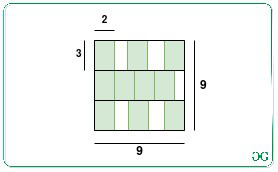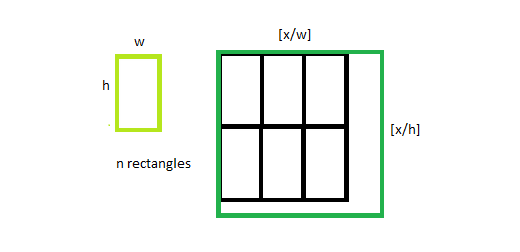GFG App
Open AppBrowser
Continue

# Size of smallest square that contains N non-overlapping rectangles of given dimensions

Given two positive integers W and H and N rectangles of dimension W*H, the task is to find the smallest size of the square required such that all the N rectangles can be packed without overlapping.

Examples:

Input: N = 10, W = 2, H = 3
Output: 9
Explanation:
The smallest size of the square is 9 units to pack the given 10 rectangles of size 2*3 as illustrated in the below image:Input: N = 1, W = 3, H = 3
Output: 3

Approach: The given problem is based on the following observations:

• It can be shown that one of the optimal spacing of rectangles within a square is given by:• The maximum number of rectangles of dimension W*H, that can be fitted in the square with sides X is given by ⌊X/W⌋⋅⌊X/H⌋.
• The above function is monotonically increasing. Therefore, the idea is to use the Binary Search to find the smallest side of a square that satisfies the given condition.

Follow the steps below to solve the problem:

• Initialize two variables, say low as 1, and high as W*H*N.
• Iterate until i is less than j and perform the following steps:
• Find the value of mid as (i + j)/2.
• Now, if the value (mid/W)*(mid/H) is at most N, then update the value of high as mid.
• Otherwise, update the value of low as (mid + 1).
• After completing the above steps, print the value of high as the resultant value.

Below is the implementation of the above approach:

## C++

 `// CPP program for the above approach` `#include` `using` `namespace` `std;`   `// Function to check if side of square X` `// can pack all the N rectangles or not` `bool` `bound(``int` `w, ``int` `h, ``int` `N, ``int` `x)` `{` `    `  `    ``// Find the number of rectangle` `    ``// it can pack` `    ``int` `val = (x / w) * (x / h);`   `    ``// If val is atleast N,` `    ``// then return true` `    ``if` `(val >= N)` `        ``return` `true``;`   `    ``// Otherwise, return false` `    ``else` `        ``return` `false``;` `}`   `// Function to find the size of the` `// smallest square that can contain` `// N rectangles of dimensions W * H` `int` `FindSquare(``int` `N, ``int` `W, ``int` `H)` `{` `    `  `    ``// Stores the lower bound` `    ``int` `i = 1;`   `    ``// Stores the upper bound` `    ``int` `j = W * H * N;`   `    ``// Iterate until i is less than j` `    ``while` `(i < j) ` `    ``{` `        `  `        ``// Calculate the mid value` `        ``int` `mid = i + (j - i) / 2;`   `        ``// If the current size of square` `        ``// cam contain N rectangles` `        ``if` `(bound(W, H, N, mid))` `            ``j = mid;`   `        ``// Otherwise, update i` `        ``else` `            ``i = mid + 1;` `    ``}`   `    ``// Return the minimum size of the` `    ``// square required` `    ``return` `j;` `}`   `// Driver code` `int` `main()` `{` `    ``int` `W = 2;` `    ``int` `H = 3;` `    ``int` `N = 10;`   `    ``// Function Call` `    ``cout << FindSquare(N, W, H);` `}`   `// This code is contributed by ipg2016107.`

## Java

 `// Java program for the above approach` `class` `GFG{` `    `  `// Function to check if side of square X` `// can pack all the N rectangles or not` `static` `boolean` `bound(``int` `w, ``int` `h, ``int` `N, ``int` `x)` `{` `    `  `    ``// Find the number of rectangle` `    ``// it can pack` `    ``int` `val = (x / w) * (x / h);`   `    ``// If val is atleast N,` `    ``// then return true` `    ``if` `(val >= N)` `        ``return` `true``;`   `    ``// Otherwise, return false` `    ``else` `        ``return` `false``;` `}`   `// Function to find the size of the` `// smallest square that can contain` `// N rectangles of dimensions W * H` `static` `int` `FindSquare(``int` `N, ``int` `W, ``int` `H)` `{` `    `  `    ``// Stores the lower bound` `    ``int` `i = ``1``;`   `    ``// Stores the upper bound` `    ``int` `j = W * H * N;`   `    ``// Iterate until i is less than j` `    ``while` `(i < j) ` `    ``{` `        `  `        ``// Calculate the mid value` `        ``int` `mid = i + (j - i) / ``2``;`   `        ``// If the current size of square` `        ``// cam contain N rectangles` `        ``if` `(bound(W, H, N, mid))` `            ``j = mid;`   `        ``// Otherwise, update i` `        ``else` `            ``i = mid + ``1``;` `    ``}`   `    ``// Return the minimum size of the` `    ``// square required` `    ``return` `j;` `}`   `// Driver code` `public` `static` `void` `main(String[] args)` `{` `    ``int` `W = ``2``;` `    ``int` `H = ``3``;` `    ``int` `N = ``10``;`   `    ``// Function Call` `    ``System.out.print(FindSquare(N, W, H));` `}` `}`   `// This code is contributed by sk944795`

## Python3

 `# Python program for the above approach`   `# Function to check if side of square X` `# can pack all the N rectangles or not` `def` `bound(w, h, N, x):`   `    ``# Find the number of rectangle` `    ``# it can pack` `    ``val ``=` `(x``/``/``w)``*``(x``/``/``h)` `    `  `    ``# If val is atleast N,` `    ``# then return true` `    ``if``(val >``=` `N):` `        ``return` `True` `      `  `    ``# Otherwise, return false` `    ``else``:` `        ``return` `False`   `# Function to find the size of the` `# smallest square that can contain` `# N rectangles of dimensions W * H` `def` `FindSquare(N, W, H):`   `    ``# Stores the lower bound` `    ``i ``=` `1` `    `  `    ``# Stores the upper bound` `    ``j ``=` `W ``*` `H``*``N` `    `  `    ``# Iterate until i is less than j` `    ``while``(i < j):` `      `  `        ``# Calculate the mid value` `        ``mid ``=` `i ``+` `(j ``-` `i)``/``/``2`   `        ``# If the current size of square` `        ``# cam contain N rectangles` `        ``if``(bound(W, H, N, mid)):` `            ``j ``=` `mid` `        `  `        ``# Otherwise, update i` `        ``else``:` `            ``i ``=` `mid ``+` `1`   `    ``# Return the minimum size of the` `    ``# square required` `    ``return` `j`   `# Driver Code`   `W ``=` `2` `H ``=` `3` `N ``=` `10`   `# Function Call` `print``(FindSquare(N, W, H))`

## C#

 `// C# program for the above approach` `using` `System;`   `class` `GFG{`   `// Function to check if side of square X` `// can pack all the N rectangles or not` `static` `bool` `bound(``int` `w, ``int` `h, ``int` `N, ``int` `x)` `{` `    `  `    ``// Find the number of rectangle` `    ``// it can pack` `    ``int` `val = (x / w) * (x / h);`   `    ``// If val is atleast N,` `    ``// then return true` `    ``if` `(val >= N)` `        ``return` `true``;`   `    ``// Otherwise, return false` `    ``else` `        ``return` `false``;` `}`   `// Function to find the size of the` `// smallest square that can contain` `// N rectangles of dimensions W * H` `static` `int` `FindSquare(``int` `N, ``int` `W, ``int` `H)` `{`   `    ``// Stores the lower bound` `    ``int` `i = 1;`   `    ``// Stores the upper bound` `    ``int` `j = W * H * N;`   `    ``// Iterate until i is less than j` `    ``while` `(i < j) ` `    ``{` `        `  `        ``// Calculate the mid value` `        ``int` `mid = i + (j - i) / 2;`   `        ``// If the current size of square` `        ``// cam contain N rectangles` `        ``if` `(bound(W, H, N, mid))` `            ``j = mid;`   `        ``// Otherwise, update i` `        ``else` `            ``i = mid + 1;` `    ``}` `    `  `    ``// Return the minimum size of the` `    ``// square required` `    ``return` `j;` `}`   `// Driver Code` `public` `static` `void` `Main()` `{` `    ``int` `W = 2;` `    ``int` `H = 3;` `    ``int` `N = 10;`   `    ``// Function Call` `    ``Console.WriteLine(FindSquare(N, W, H));` `}` `}`   `// This code is contributed by ukasp`

## Javascript

 ``

Output:

`9`

Time Complexity: O(log(W*H))
Auxiliary Space: O(1)

My Personal Notes arrow_drop_up